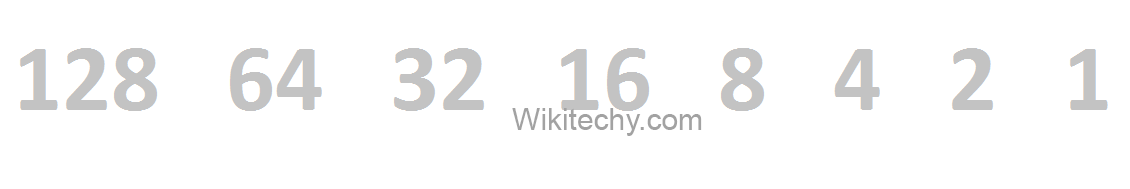# Decimal C# | Convert Decimal to Binary | C# Program to Convert Decimal to Binary - c# - c# tutorial - c# net

## Decimal Number

• Decimal number is a base 10 number because it ranges from 0 to 9, there are total 10 digits between 0 to 9. Any combination of digits is decimal number such as 223, 585, 192, 0, 7 etc.

## Binary Number

• Binary number is a base 2 number because it is either 0 or 1. Any combination of 0 and 1 is binary number such as 1001, 101, 11111, 101010 etc.
• Let's see some binary numbers for the decimal number.
Decimal Binary
1 0
2 10
3 11
4 100
5 101
6 110
7 111
8 1000
9 1001
10 1010Convert Decimal to Binary in C#

## Decimal to Binary Conversion Algorithm

• Step 1: Divide the number by 2 through % (modulus operator) and store the remainder in array
• Step 2: Divide the number by 2 through / (division operator)
• Step 3: Repeat the step 2 until the number is greater than zero
• Let's see the C# example to convert decimal to binary.